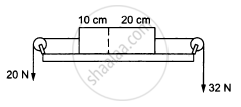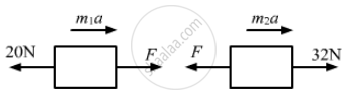# Figure (5−E8) Shows a Uniform Rod of Length 30 Cm and Mass 3.0 Kg. the Strings Shown in the Figure Are Pulled by Constant Forces of 20 N and 32 N. - Physics

Sum

In the following figure shows a uniform rod of length 30 cm and mass 3.0 kg. The strings shown in the figure are pulled by constant forces of 20 N and 32 N. Find the force exerted by the 20 cm part of the rod on the 10 cm part. All the surfaces are smooth and the strings and the pulleys are light.#### Solution

Mass per unit length $= \frac{3}{30} kg/cm$ = 0.10 kg/cm
Mass of the 10 cm part, m1 = 1 kg
Mass of the 20 cm part, m2 = 2 kg

Let F = contact force between them.From the free-body diagram,
$m_1 a = F - 20 . . . \left( i \right)$
$m_2 a = 32 - F . . . \left( ii \right)$
$\text{ Adding both the equations, we get: }$
$a = \frac{12}{m_1 + m_2} = \frac{12}{3} = 4 m/ s^2$
So, contact force,
F = 20 + 1a
F = 20 + 4 = 24 N

Concept: Newton’s Second Law of Motion
Is there an error in this question or solution?

#### APPEARS IN

HC Verma Class 11, Class 12 Concepts of Physics Vol. 1
Chapter 5 Newton's Laws of Motion
Q 24 | Page 80